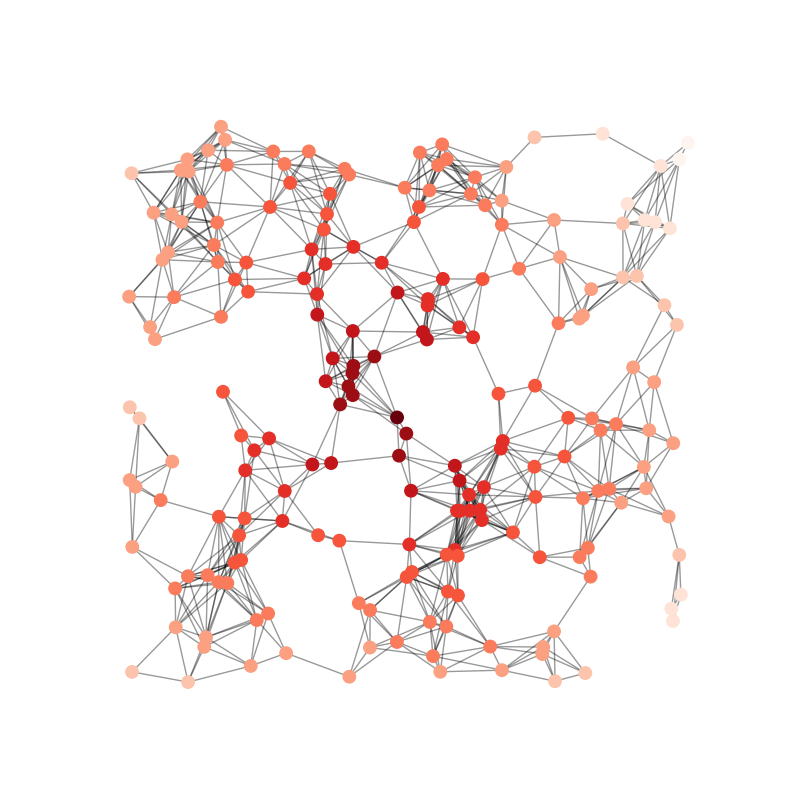# Random Geometric Graph#

Example```import matplotlib.pyplot as plt
import networkx as nx

# Use seed when creating the graph for reproducibility
G = nx.random_geometric_graph(200, 0.125, seed=896803)
# position is stored as node attribute data for random_geometric_graph
pos = nx.get_node_attributes(G, "pos")

# find node near center (0.5,0.5)
dmin = 1
ncenter = 0
for n in pos:
x, y = pos[n]
d = (x - 0.5) ** 2 + (y - 0.5) ** 2
if d < dmin:
ncenter = n
dmin = d

# color by path length from node near center
p = dict(nx.single_source_shortest_path_length(G, ncenter))

plt.figure(figsize=(8, 8))
nx.draw_networkx_edges(G, pos, alpha=0.4)
nx.draw_networkx_nodes(
G,
pos,
nodelist=list(p.keys()),
node_size=80,
node_color=list(p.values()),
cmap=plt.cm.Reds_r,
)

plt.xlim(-0.05, 1.05)
plt.ylim(-0.05, 1.05)
plt.axis("off")
plt.show()
```

Total running time of the script: (0 minutes 0.091 seconds)

Gallery generated by Sphinx-Gallery